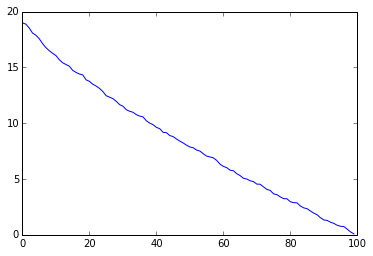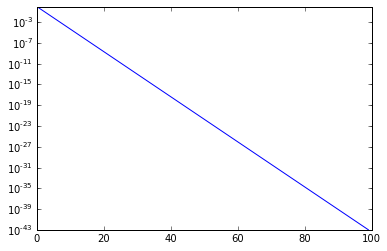# Randomized SVD

In :
import numpy as np
import numpy.linalg as la
import matplotlib.pyplot as pt


## Obtaining a low-rank matrix

Let's begin by making a low-rank matrix:

In :
n = 100
A0 = np.random.randn(n, n)
U0, sigma0, VT0 = la.svd(A0)
print(la.norm((U0*sigma0).dot(VT0) - A0))

pt.plot(sigma0)

2.67019320578e-13


Out:
[<matplotlib.lines.Line2D at 0x7fd35095af98>]A randomly drawn Gaussian matrix: Emphatically not low-rank. Let's swap out the singular values with cleanly exponentially decaying ones:

In :
sigma = np.exp(-np.arange(n))
pt.semilogy(sigma)

A = (U0 * sigma).dot(VT0)## Find the approximate range

Let's fix parameters first. What accuracy should we obtain for the values of $k$ and $p$ below? (where p represents the 'extra' dimensions)

In :
k = 10
p = 5


Draw a random Gaussian matrix Omega and obtain orthogonal columns in a matrix Q spanning the range:

In :
Omega = np.random.randn(n, k+p)
Y = A.dot(Omega)
Q, _ = la.qr(Y)


As an alternative to the above, use a few iterations of the power method on Omega:

In :
Omega = np.random.randn(n, k+p)
Y = A.dot(Omega)
Y = A.dot(A.T.dot(Y))
Q, _ = la.qr(Y)


## Convert to factorization form

Reconstruct $C$ in the factorization-form LRA $A\approx BC$:

(Recall $A\approx QQ^TA$, $B=Q$)

In :
C = Q.T.dot(A)
print(C.shape)

(15, 100)



Sanity-check that form:

In :
Aapprox1 = Q.dot(C)
la.norm(A - Aapprox1, 2)

Out:
3.9724863710473977e-06


## Compute the approximate SVD

Compute the SVD of $C=U_C \Sigma_C V_C^T$:

(Make sure to pass full_matrices=False to the SVD.)

In :
UC, sigmaC, VTC = la.svd(C, full_matrices=False)


Reconstruct the SVD of $A$: $A\approx QU_C \Sigma_C V_C^T$

In :
UAapprox = Q.dot(UC)
sigmaAapprox = sigmaC
VTAapprox = VTC


Compare the 2-norm of the reconstructed $A$ with the original $A$:

In :
Aapprox = (UAapprox*sigmaAapprox).dot(VTAapprox)
la.norm(A - Aapprox, 2)

Out:
3.972486371045497e-06


## Estimate the error

Compute an a-posteriori estimate of approximation error in the spectral norm:

In :
omega = np.random.randn(n)
Aomega = A.dot(omega)
err = Aomega - Q.dot(Q.T.dot(Aomega))
la.norm(err, 2) / la.norm(omega, 2)

Out:
3.3931213029085818e-07

• Is this the right direction for the error estimator?
• Is the estimator supposed to be conservative? Can it be?